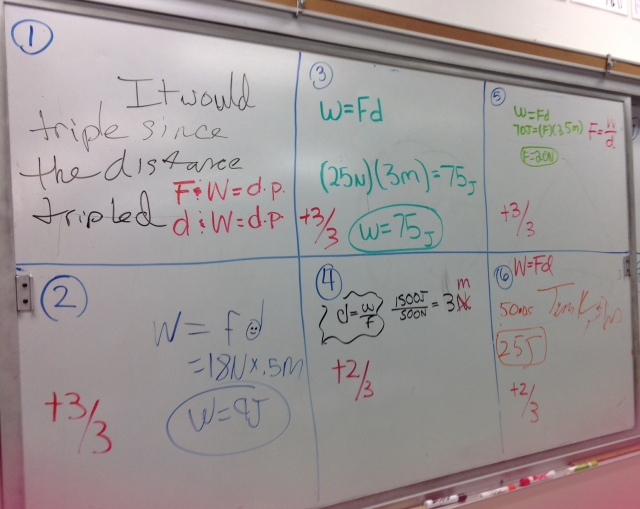# What is Work?

12 teachers like this lesson
Print Lesson

## Objective

Students will be able to define work, describe how it is related to force and distance, and calculate work.

#### Big Idea

Students learn and apply the concept of work.

## First Look at Energy Unit Learning Targets

10 minutes

The goal of this lesson is to introduce students to work. Students will use their computational thinking to apply ideas of work to various calculations (SP5) as well as share answers with the class through the whiteboard session (SP8). They learn about work first so that students can build upon their knowledge and eventually to energy conservation (HS-PS3-1).

To begin class, I pass out the Unit 5 Energy Learning Target Sheet and calendar for the unit to students. I give students the learning targets so they can see exactly what we are going to cover over the course of the unit. I give them the calendar so students can see what the homework assignments will be and when the major assessments will be so they can plan their study and work time accordingly.

At the beginning of every unit we do a first look at the targets as a preview to the unit. I ask students to read through all of the targets and rate on a scale of 1-4 how they feel about their knowledge and ability to complete that learning target. We use the Assess Yourself labels of (1) Novice, (2) Apprentice, (3) Practitioner, and (4) Expert. I ask students to silently read through the targets and rate themselves on the targets using a symbol the class chooses (ex. circle, square, star, smiley face). When all students read through all of the targets and rate themselves on the learning targets, I tell them that we will do a couple of checks along the way to see how they are doing with each target throughout the unit.

## Work Guided Notes

15 minutes

After the students have read through the learning targets, we focus our attention as a class on the first two learning targets regarding work. I use the Work Guided Notes as a template to provide information to students. I like to use guided notes because it helps students to focus better during notes on the important information. If students are absent, I have a video that takes them through the guided notes step-by-step, as shown below.

As we go through the notes, I define work and talk about the relationship between force, distance and work. After we discover the equation to solve for work, I emphasize the units and how the Newtons and meters give us Joules. Before completing some calculations with the new equation, I go through some situational examples of work and some situational non-examples of work.

To end the guided notes, I go through two examples. The first example is pretty straight forward providing a force and a distance to calculate work. The second example requires students to convert the mass (in kg) to weight, a force, (in N) before putting it into the work equation. I like to give them an example of each prior to working on a problem set so they have a template in how to solve problems.

## Work Problem Set

20 minutes

After the guided notes, I have students work on the Work Problem WS. I ask them to show all of their work similar to the notes that we took. I have them work with their table groups to complete the problems. This time is meant to be practice so that they can use what they have learned from the guided notes. I tell students that students will be putting their answers up in a whiteboard session to review the answers, but each group must complete all of the problems before the session. I make sure that students complete their work by randomly selecting one students paper to scan through to see if the group completed the problems.

## Mega-Whiteboard Session

10 minutes

After students complete the work problem set, I ask them to volunteer to write their solutions on the board. I require that at least one person from each of the 7 tables comes to the front; I then allow additional students from the same table to go up. I have students show all of their work on the board. When all student volunteers have put their answers up, I review the problems, 1 at a time to see if there are any questions. As I go through each problem, I grade the problem on the board as I would grade it on a quiz or test (out of 3 points). I go over the problems this way because it is a quick way and allows students to check their answers to see how they did. The students work is shown below after we went over it as a class.## Work Checkpoint

5 minutes

To end class, I have students complete a checkpoint to see what they have learned from the lesson. Students complete the Work Checkpoint individually. I ask them to determine if work is being done in a situation based on a description and, if work is done, to calculate the amount of work done on an object. I use this activity to see if there is anything that I need to go over again before moving on to other topics like power, energy and energy conservation. I grade these and return to the students at the beginning of the next class.

For homework, students watch the power guided notes video and complete the Power Guided Notes page. I do this as a flipped classroom model because I want students to be able to spend more time in class working with the problems and doing other activities that extend their thinking.